# Cube in a sphere

The cube is inscribed in a sphere with volume 7253 cm3. Determine the length of the edges of a cube.

Result

a =  13.9 cm

#### Solution:

$V=7253 \ \text{cm}^3 \ \\ V=\dfrac{ 4 }{ 3 } \pi r^3 \ \\ \ \\ r=\sqrt{ \dfrac{ 3 \cdot \ V }{ 4 \pi } }=\sqrt{ \dfrac{ 3 \cdot \ 7253 }{ 4 \cdot \ 3.1416 } } \doteq 12.0082 \ \text{cm} \ \\ \ \\ D=2 \cdot \ r=2 \cdot \ 12.0082 \doteq 24.0163 \ \text{cm} \ \\ \ \\ u=D=24.0163 \doteq 24.0163 \ \text{cm} \ \\ \ \\ u=\sqrt{ 3 } a \ \\ \ \\ a=u/\sqrt{ 3 }=24.0163/\sqrt{ 3 } \doteq 13.8658 \doteq 13.9 \ \text{cm}$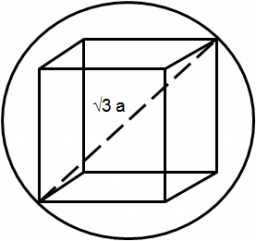Our examples were largely sent or created by pupils and students themselves. Therefore, we would be pleased if you could send us any errors you found, spelling mistakes, or rephasing the example. Thank you!

Leave us a comment of this math problem and its solution (i.e. if it is still somewhat unclear...):Math student
i am good at this

2 years ago  2 LikesCrazy Butterfly
This is easy.Tips to related online calculators
Need help calculate sum, simplify or multiply fractions? Try our fraction calculator.
Do you want to convert length units?
Tip: Our volume units converter will help you with the conversion of volume units.
Pythagorean theorem is the base for the right triangle calculator.

## Next similar math problems:

1. Wall thicknessThe hollow metal ball has an outside diameter of 40 cm. Determine the wall thickness if the weight is 25 kg and the metal density is 8.45 g/cm3.
2. Two rectangular boxesTwo rectangular boxes with dimensions of 5 cm, 8 cm, 10 cm, and 5 cm, 12 cm, 1 dm are to be replaced by a single cube box of the same cubic volume. Calculate its surface.
3. Cuboid and ratioFind the dimensions of a cuboid having a volume of 810 cm3 if the lengths of its edges coming from the same vertex are in ratio 2: 3: 5
4. The largest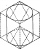The largest possible cylinder was cut from a 20 cm cube. What is the volume of this cylinder?
5. The edge of a cube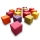How much does the edge of a cube of 54.9 cm3 measure?
6. Surface of the cubeFind the surface of the cube that has volume 1/1m3 2/0.001 m3 3/8000 mm3
7. Nádoba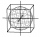Nádoba tvaru kostky je naplněna vodou do poloviny své výšky. Pokud dolijeme 20 l vody, bude nádoba naplněna do tří čtvrtin své výšky. Jaký je objem celé nádoby?
8. Hemisphere - roof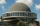The shape of the observatory dome is close to the hemisphere. Its outer diameter is 11 m. How many kilograms of paint and how many liters of paint is used for its double coat if you know that 1 kg of paint diluted with 1 deciliter of paint will paint an a
9. Triangular prismThe base of the perpendicular triangular prism is a rectangular triangle with a hypotenuse of 10 cm and one leg of 8 cm. The prism height is 75% of the perimeter of the base. Calculate the volume and surface of the prism.
10. Half-filled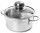A cylindrical pot with a diameter of 24 cm is half-filled with water. How many centimeters will the level rise if we add a liter of water to it?
11. Tetrahedral pyramid 8Let’s all side edges of the tetrahedral pyramid ABCDV be equally long and its base let’s be a rectangle. Determine its volume if you know the deviations A=40° B=70° of the planes of adjacent sidewalls and the plane of the base and the height h=16 of the p
12. Block or cuboidThe wall diagonals of the block have sizes of √29cm, √34cm, √13cm. Calculate the surface and volume of the block.Calculate the volume (V) and the surface (S) of a regular quadrilateral prism whose height is 28.6 cm and the deviation of the body diagonal from the base plane is 50°.The regular triangular prism, whose edges are identical, has a surface of 2514 cm ^ 2 (square). Find the volume of this body in cm3 (l).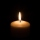A company makes wax candles in the shape of a cylinder. Each candle has a diameter of 4 inches and a height of 7 inches. If the company used 7561.12 in3 of wax, how many candles did it make? Use 3.14, and do not round your answerThe cylinder volume is 150 dm cubic, the base diameter is 100 cm. What is the height of the cylinder?Try to estimate the weight of the water in an aquarium 50cm long 30cm wide, when poured to a height of 25cm. Calculates the weight of the aquarium's water.